Ch 12. Infinite Sequences and Series Multimedia Engineering Math Sequencesand Series Integral andComparison Alt. Series &Abs. Conv. PowerSeries Taylor, Mac. &Binomial
 Chapter 1. Limits 2. Derivatives I 3. Derivatives II 4. Mean Value 5. Curve Sketching 6. Integrals 7. Inverse Functions 8. Integration Tech. 9. Integrate App. 10. Parametric Eqs. 11. Polar Coord. 12. Series Appendix Basic Math Units Search eBooks Dynamics Fluids Math Mechanics Statics Thermodynamics Author(s): Hengzhong Wen Chean Chin Ngo Meirong Huang Kurt Gramoll ©Kurt GramollMATHEMATICS - THEORY The concept of series was introduced in the previous section, and it is known that a series is convergent when it has a finite sum. Two more convergence tests, the integral test and the comparison test, will be presented in this section. Integral Test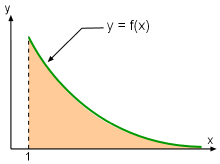Improper Integral As mentioned in the previous section, the total sum of a series is often desired, but it is not trivial to obtain. The knowledge of whether a series is convergent or divergent becomes important since there is no point trying to determine the total sum of a series if it is a divergent series. The integral test is one of the methods which can be used to determine the convergence of a series, and it is stated as follows: Let f be a positive, continuous, and decreasing function on [1,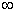) and an = f(n), then (1) The series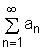is convergent if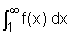is convergent. (2) The seriesis divergent ifis divergent. Note that the improper integral is involved in the integral test. Furthermore, if one uses a partial sum (Sn) to estimate the total sum (S) of a series using the integral test, the remainder Rn = S - Sn is given by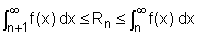The above inequalities can be further refined by adding Sn to both sides to yield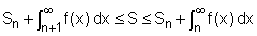where S = Rn + Sn. These inequalities give a lower bound and upper bound for the estimation of the total sum of a given series. It is a common practice to take the midpoint of the interval for the approximation, and it provides a more accurate estimation. For example, take the series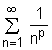(also known as the p-series). Let f(x) = 1/xp, and it is a positive, continuous and decreasing function. Applying the integral test to this series yields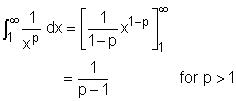Hence, the p-series converges if p > 1. Comparison Test The comparison test is another method to determine if a non-negative series is convergent or divergent. The basic idea of the comparison test is to compare a series with another series that is known to be convergent or divergent. The comparison test is formally stated as follows: Let Σan and Σbn be series with positive terms. (1) If Σbn is convergent and an ≤ bn for all n, then Σan is convergent. (2) If Σbn is divergent and an ≥ bn for all n, then Σan is divergent. The above test is also referred to as the basic comparison test. Sometimes, the basic comparison test is difficult to apply. A more useful version is the limit comparison test, which is stated as follows: Let Σan and Σbn be series with positive terms. (1) If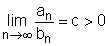, then either both series converge or both diverge. (2) If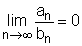and Σbn is convergent, then Σan is convergent. (3) If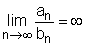and Σbn is divergent, then Σan is divergent.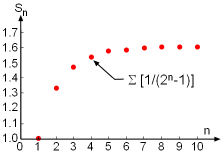The Partial Sum of the Series Σ(2n - 1) Subject to Different n Values. Now, let's use the limit comparison test to determine if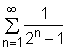converges or diverges. Let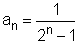and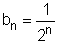where bn is a convergent geometric series. Applying the limit comparison test yields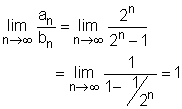Hence, the given series is convergent. The partial sum of this given series subject to different n values is shown in the figure, and it confirms that the series is convergent.